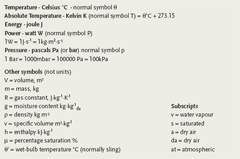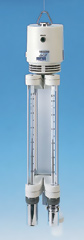# Module 7: Applying the psychrometric relationships

The CPD article in the April edition of CIBSE Journal considered the majority of the properties of moist air that may be determined from a pair of coincident properties using the psychrometric chart. This article will continue to develop the formulae that may be used to calculate the properties and consider the comparative accuracy of using a chart compared with simple calculations

Arguably the one process that determines whether a system is air conditioning, as opposed to simply ventilation, is the control of air moisture content. So a key requirement of psychrometric analysis is the ability to establish the vapour pressure that in turn can be used to calculate the air moisture content.

To establish the vapour pressure in air, pv(kPa) the relationship based on Sprung’s original experiments with in the late 19th century known as the psychrometric equation may be used

pV = ps’ – pat A(θ – θ’)

where, for a sling wet-bulb temperature,

A = 6.66×10-4K-1 where θ’ ≥ 0 deg C

A = 5.94×10-4K-1 where θ’ < 0 deg C

Where ps’ is the saturation vapour pressure at the wet-bulb temperature that can be determined from tables or from the rather lengthy but suitably accurate empirical equation that is used to determine saturation vapour pressure, ps (kPa)

log ps = 30.5905 – 8.2 log (θ + 273.16) + 2.4804 x 10–3 (θ + 273.16) – [3142.31 / (θ + 273.16)]

and this is ideally suited to use in a spreadsheet or web tool (and is valid for temperatures above 0 deg C). Note that the saturation vapour pressure is not dependent upon the atmospheric pressure.

### The final volume of the psychrometric story?

An element of the standard psychrometric chart that causes widespread uncertainty is the specific volume and more particularly its relationship with density. Many texts simply relate one as the inverse of the other – however there is a convention in the HVAC&R world that, for humid air, this is not the case.

The specific volume of moist air, v (m3·kg-1da), is the volume of 1 kg dry air and its associated water vapour, so using the gas law pV = mRT,

v = V/ma = RaT/pa = RaT /(pat – pv)

= 287·T/(pat – pv)

where V is the volume occupied by the moist air, ma is the mass of the dry air (that is combined with the mass of water vapour, mv, to give [ma + mv] kg moist air), pa is dry air partial pressure, pv is water vapour partial pressure and pat is atmospheric pressure.

Density, ρ (kg·m-3), is the mass of moist air that occupies a volume of 1 m3. To evaluate the mass of 1 m3 of moist air the masses of the dry air and the water vapour are added together.

So as ma/V=pa/RaT and mv/V=pv/RvT the overall density of the single unit volume of moist air will be (pa/RaT + pv/RvT).

Rearranging this and putting in values for Ra and Rv gives

ρ = (pat/287·T)[1-(0.378·pv/pat)] kg·m-3

(with all pressures in Pa)

As an example consider air at 20.0 deg C dry bulb and 13.9 deg C wet bulb at an atmospheric pressure of 101.325 kPa = 101325Pa (ie standard atmospheric pressure).

To find the vapour pressure

pv = ps’ – pat A (θ – θ’)

a value of ps’ is required and so using…

log ps’ = 30.5905 – 8.2 log (13.9 + 273.16) + 2.4804 x 10-3 (13.9 + 273.16) – [3142.31 / (13.9 + 273.16)]

and so ps’ = 1.591 kPa.

Hence

pv = 1.591 – [101.325 x 6.66 x 10-4 x (20 – 13.9)] = 1.179kPa

And so to determine v and ρ

v = 287·T / (pat – pv) = 287 (273+20)/(101325 – 1179) = 0.839 m3·kg-1

ρ = (pat/287·T)[1-(0.378·pv/pat)] = [101325/(287 x (273+20))][1-(0.378 x 1179/101325)] = 1.200 kg·m-3

And to see what the practical difference is between the inverse of specific volume and density (at this particular condition) consider that the reciprocal of the specific volume calculated above is 1.192 and this compares with a density of 1.200 – a difference of less than 1 per cent. The difference will rise as moisture content increases and in conditions that are likely to prevail in heating, ventilation and air conditioning applications there is likely to be maximum of about 4 per cent difference at high temperatures and high moisture contents between the simple reciprocal value of specific volume and the true density.

Specific volume is particularly useful when undertaking calculations where there is a change in moisture content during a process (humidification and dehumidification), as the amount of dry air will remain constant while the amount of water vapour changes. However density is useful when measuring the flowrate of air through pressure drop devices (such as orifice plates).

Considering the formula for air density above, moist air is less dense than dry air. This may seem counter intuitive however the ideal gas law states that a fixed volume of air at a certain pressure has a fixed number of molecules and each of those molecules has a particular mass. Looking back at the previous article dry air is made up principally of nitrogen (N2) and oxygen (O2) molecules with molar masses of 28 and 32 respectively. Water vapour has a molar mass of 18. Hence, when a given volume of air has water molecules displacing other heavier molecules its overall mass and hence density will be less.

### Plotting the air point

Using the relationships described in this, and the previous, article and using nothing more than a sling psychrometer and a psychrometric chart or a hand held calculator (mobile phone, palmtop etc) the properties of air may readily be obtained.Figure 1: Plotting psychrometric propertiesUnit guide

As an example the air point of 20 deg C dry-bulb and 13.9 deg C wet-bulb (sling) is shown in Figure 1. The dry-bulb and wetbulb temperatures may well have been obtained using a sling psychrometer in a room – the standard chart is drawn up for an atmospheric pressure, pat, of 101.325kPa. The other properties may then be read directly off the chart with the specific enthalpy obtained by aligning a straight edge through the plotted air point so that the same value is read on both the ‘laddered’ specific enthalpy scales (shown with the black dashed line in Figure 1).

These values may also be calculated. So using the values obtained from the earlier example for air at θ = 20.0°C and θ’=13.9°C.

v was calculated as 0.839 m3·kg-1 (compares with 0.84 m3·kg-1 from the chart)

pv is 1.179kPa (previously calculated)

and so

g = 0.622 pv/(pat – pv) = 0.622 x 1.179/(1013.25-1.179) = 0.0073 kg·kg-1 (0.0074 kg/kg from chart)

To determine the percentage saturation the value of the saturated moisture content at the dry bulb temperature of 20 deg C is required. This can be obtained using the formula for g but using saturated vapour pressure (at the dry bulb temperature), ps, in place of pv to determine gs. And ps (at 20 deg C) can be obtained from

log ps = 30.5905 – 8.2 log (20 + 273.16) + 2.4804 x 10-3 (20 + 273.16) – [3142.31 / (20 + 273.16)] and so ps = 2.342 kPa.

gs = 0.622 ps/(pat – ps) = 0.622 x 2.342/(1013.25-2.342) = 0.0147 kg·kg-1

μ = g/gs x 100 = (0.0073/0.0147) x 100 = 50 per cent (compares with 50 per cent on chart)

Finally the Specific Enthalpy, h may be determined from

h = ( 1.005 θ) + g (1.89 θ +2501) = (1.005 x 20) + 0.0073 (1.89 x 20 + 2501) = 39 kJ·kg-1 (the same value as on chart).

### Psychrometric accuracy

There is evidently reasonable correlation between reading information off the chart and the calculated values and this should provide some confidence (and will be similarly accurate across the whole chart). However it is important to keep in mind that the accuracy of the output data can be no better than the measurements that have been taken on-site or, perhaps, read from a screen of a building management system (BMS). The perception may be that since, for example, a BMS output screen shows several significant figures that the measurement is precise. This is unlikely to be the case. Legg reports that the practical uncertainties in the measurement of wet-bulb temperatures can lead to significant uncertainties.

The wet-bulb sensor (whether a true wet-bulb or electronic sensor) is susceptible to contamination by both air borne contamination as well as finger grease. The wetted wick on a wet-bulb needs to stabilise at the wet bulb temperature before the temperature may be recorded. Tap water or other impure water can cause measurement errors when used to wet the wick, and if the wick (covering the bulb of a wet-bulb thermometer) is too long it will be affected by heat conducted from the thermometer.

Small variations in recorded temperature can translate into significant variations in percentage saturation. For example the gradations on the stem of a thermometer are likely to be 0.5 deg C – this alone will lead to an uncertainty of +/-2.5 per cent in the derived percentage saturation. To reduce possibilities of operator error devices such as the Assmann Psychrometer may be used to measure room air conditions (see Figure 2) – they have finer gradations on the temperature scales and provide a more consistent method of measurement with air being drawn over the thermometers by a small motor driven fan. This ensures that the air velocity is drawn across the wet bulb at the minimum required 1m·s-1.Figure 2: Assmann Psychrometer (source: www.mesdan.com)

In terms of practical application of the psychrometric chart it has been observed that many seasoned users prefer to use a thicker pen rather than a sharp pencil when plotting lines and identifying air points, as it builds in a clearly visible tolerance, or uncertainty, that reflects the real world challenge in obtaining an ‘accurate’ measurement.

A future article will apply the Psychrometric Chart to processes and systems.